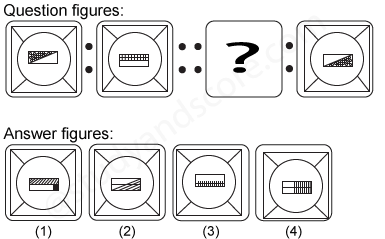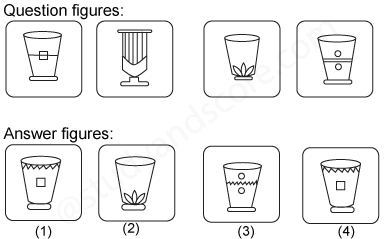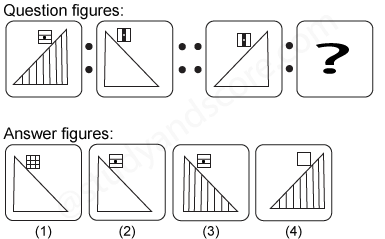# Analogy- Test 3

1)

Select the related figure from the given answer figures.

[SSC CGL, 2005]2)

Select the related figure from the given answer figures.

[SSC CGL, 2005]3)

Four pairs of flower pots are given below. Among these pairs only one pair is similar in all respects. Identify the pair

[SSC CGL, 2005]4)

Select the related figure from the given answer figures.

[SSC CGL, 2005]5)

Select the related figure from the given answer figures.

[SSC CGL, 2005]--Share with your friends --